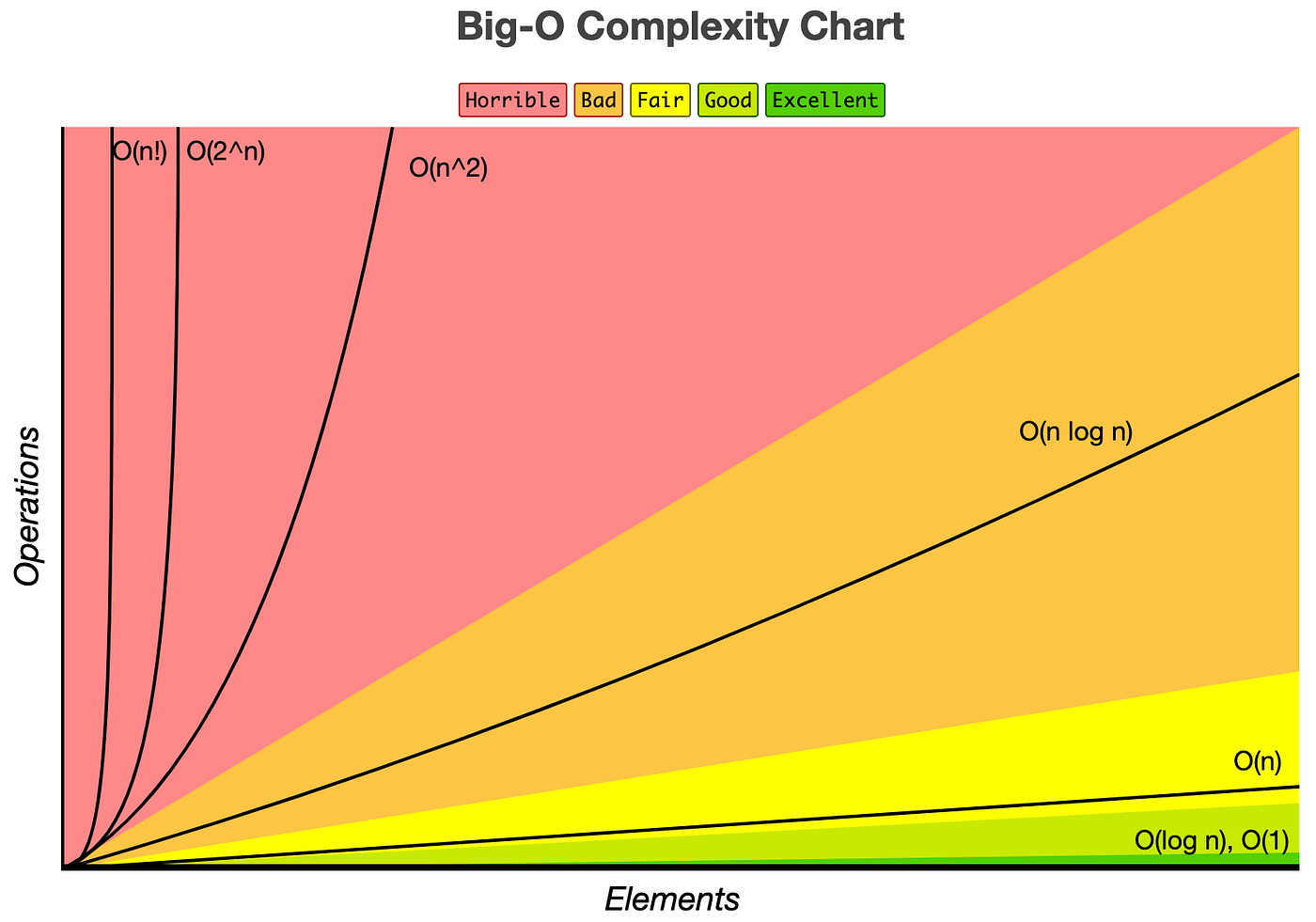# Day 8 - 基礎計概 & 演算法

### 進位制度 Positional notation

• 十進位 Decimal (簡寫 dec)
• 二進位 Binary
• 八進位 Octal (簡寫 oct)

#### 電腦裡的色碼表示

##### 範例

R: `FF` , G: `FF`, B: `00`，所以色碼是 `#FFFF00`#### 二進位 Binary

##### `56`（十進位）轉換成 `111000`（二進位）的思路：
1. 56 和 2 的哪個次方最接近？答 `2^5 = 32`
2. 56 減去 32 剩下 24
3. 對 24 重複 Step 1. & 2. 的處理方式...
4. 最後可知 `56 = 2^5 + 2^4 + 2^3`
5. 再寫得更仔細 56 = `1` * 2^5 + `1` * 2^4 + `1` * 2^3 + `0` * 2^2 + `0` * 2^1 + `0` * 2^0
6. 所以換到二進位 `111000`
##### 其他練習

27 = 2^4 + 2^3 + 2^1 + 2^0 -> 11011
32 = 2^5 -> 100000
100 = 2^6 + 2^5 + 2^2 -> 1100100

#### 二位元負數

##### 舉例
``````0   1   1   1   1   1   1   1   =   127
0   0   0   0   0   0   1   0   =   2
0   0   0   0   0   0   0   1   =   1
0   0   0   0   0   0   0   0   =   0
1   1   1   1   1   1   1   1   =   −1
1   1   1   1   1   1   1   0   =   −2
1   0   0   0   0   0   0   1   =   −127
1   0   0   0   0   0   0   0   =   −128
``````

#### 浮點數[C&C++] 浮點數精準度 (Floating-Point Precision)

### 儲存空間單位

• 1 Byte = 8 Bits，Byte 又稱位元組
• 1 Kilobyte (KB) = 1024 Bytes
• 1 Megabyte (MB) = 1024 KB
• 1 Gigabyte (GB) = 1024 MB
• 1 Terabyte (TB) = 1024 GB
• 1 Petabyte (PB) = 1024 TB
• 1 Exabyte (EB) = 1024 PB
• 1 Zettabyte (ZB) = 1024 EB
• 1 Yottabyte (YB) = 1024 ZB

### 演算法 Algorithm

##### 輸入 + 演算法 = 輸出

`複雜度` 來衡量演算法的好壞，分兩類

• 時間複雜度（Time Complexity）：演算法需要消耗的時間資源。
• 空間複雜度（Space Complexity）：演算法需要消耗的空間資源。

#### 時間複雜度（Time Complexity）#### 排序演算法（Sorting algorithm）

##### 選擇排序法（Selection sort）
1. 首先在未排序序列中找到最小（大）元素，存放到排序序列的起始位置。
2. 再從剩餘未排序元素中繼續尋找最小（大）元素，然後放到已排序序列的末尾。
3. 以此類推，直到所有元素均排序完畢。
##### 泡沫排序法（Bubble sort）
1. 從序列第一個元素開始往後比較。
2. 若後面的比現在挑中的還大，那就把挑中的元素換成該數繼續往後比；若後面的比現在挑中的還小，那就把兩者順序對調然後再和下一個數字比。
3. 重複以上步驟直到排序結束。

##### 合併排序
1. 將陣列分割直到只有一個元素。
2. 開始兩兩合併，每次合併同時進行排序，合併出排序過的陣列。
3. 重複 2 的動作直接全部合併完成。
##### 快速排序
1. 挑選基準值：從數列中挑出一個元素，稱為「基準」（pivot），
2. 分割：重新排序數列，所有比基準值小的元素擺放在基準前面，所有比基準值大的元素擺在基準後面（與基準值相等的數可以到任何一邊）。在這個分割結束之後，對基準值的排序就已經完成，
3. 遞迴排序子序列：遞迴地將小於基準值元素的子序列和大於基準值元素的子序列排序。
#Computer #計算機概論 #演算法 #二進位 #learning